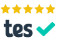# OCR A level maths H240 2018 paper 3: interactive! (with mouse)

### Hover the mouse over a vector for guidance.ARecommended Resource

Main page - lots of interactive A level mechanics

9.(i) The two vertical reaction forces must sum to equal the vertical weight of the plank.

(ii) Drag the upper group of three rotatable moments so their join is at A. (Hover the mouse near but not quite where the stems join, then left-click and drag.) See how equilibrium means that the two anti-clockwise moments created by the reactions at C and D must equal the clockwise one created by the weight of the plank acting at the centre of mass half way along. Solve for x.

(iii) Move the moments away from A. Move the other pair so their join is at D. Since the plank is going to tilt about D there is no reaction at C. So the only two moments are created by the weights of the plank, at the centre, which we now know is x + 0.5 - 2 = 0.375 from D, and of the stone block at B, which is 2 - 0.375 = 1.625 from D. Equilibrium means these must be equal, so you can solve for the weight of the stone block at B.

Your browser does not support the HTML5 canvas tag.

load a saved work file or a background image file:

10.

(i) Drag (hover over a vector near to but not on the join, then click and drag) the two vectors on the right onto the picture, or drag the picture on the vectors, to see how we are trying to match the horizontal components with each other. One is 4 sin(2 theta) and the other is 6 sin theta, and these must be equal because they are the only horizontal forces and the system is in equilibrium. So we just solve for theta.

(ii) Similarly, the two (upward) vertical components must sum to a total which is equal to the downward force.

(iii) Now (with the 4N force removed) the net total of all the vertical forces will be the downward force of 5N minus the upward component (cos theta) of 3N. And now there is only one horizontal force to consider. Apply Pythagoras for the magnitude of the resultant and inverse tan for its direction relative to the horizontal. (Or the vertical. Be sure to declare which.)

Your browser does not support the HTML5 canvas tag.

load a saved work file or a background image file:

11.

(i) Differentiate v to give a = k + 0.06t, e.g. 1.3 = k + 0.06(20).

(ii) So the velocity at 20 seconds = 0.1(20) + 0.03(20)2. Then integrate to find the two areas under the graph: before and after 20 seconds. Constant acceleration for the second area allows use of a 'suvat', e.g. v2 = u2 + 2as.

Your browser does not support the HTML5 canvas tag.

load a saved work file or a background image file:

12.

(i) Starting at B, on which only two equal forces are acting, we see that T, the tension, is equal to the weight, one quarter mg. At A, we should first just collect all the forces acting and consider their directions. Two and a half of them (so to speak) are parallel to the slope, this being the best direction in which to resolve: tension T, friction F (note that it is acting up the slope), and then a component of the weight.

Hover the mouse near the join of the three vectors far right - so that a grey sausage shape (not a circle) is visible - then left click and drag the whole shape so that the join is on A. Now click on the grey circle at the join and drag to play around. For example drag the join so that the initially vertical red (resultant) arrow is horizontal. Try to see how the "big and little" angles in the right triangle in the diagram show you how to draw a vector parallelogram which is correct just because "big and little" correspond appropriately.

Now press control-Z until the display is back to how it was. See how equilibrium means that T and F must total the same as the component of mg which is parallel to the slope. See how this must be a half of mg because of the 30-60 trigonometry. (Click near the end of a vector to get dotted lines completing the triangles, which may help to see the trigonometry.)

So now we can make an equation with T, F and mg. With T equal to a quarter mg, and F equal to μ times R, where R in turn is equal to the other component of the weight, acting perpendicular to the slope, you can follow the algebra to its conclusion as shown.

For (b) set μ to the minimum of zero, to find the value of λ in that case.

(ii) This time the equations for A and B will be equations of accelerating motion. So, starting with B again, see how the "ma", the force (or rate of change of momentum) with which the particle is moving must be equal to its weight minus the retarding force of tension.

Over at A (friction now acting down the slope), the ma must be equal to the tension pulling it minus the retarding forces of friction and a component of the weight.

Making T the subject of each equation will facilitate its elimination, so you can solve for μ once more.

Your browser does not support the HTML5 canvas tag.

load a saved work file or a background image file:

Main page - more interactive A level mechanics

ARecommended Resource
Garden City Maths - Online tuition!Today! Open a meeting in Zoom and invite by email (below). Or just email me, and I'll help set up.
Balloon Calculus ... Don't integrate - balloontegrate!

email: tom@ballooncalculus.org.